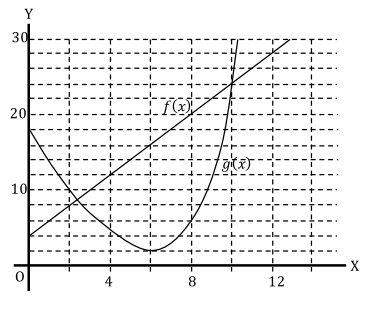# Free SAT Practice Questions

Question 1 of 1
ID: SAT-PTAM-5
Section: Math - Passport to Advanced Math
Topics: Graphic functions; Deduction of value
Difficulty level: Challenging

(Practice Mode: Single selected Question » Back to Overview)The given graph represents two functions $f\left(x\right)$ and $g\left(x\right)$. If $f\left(2\right)=k$, which of the following is the value of $g\left(k\right)$? (without calculator)

A0
B2
C4
D8